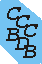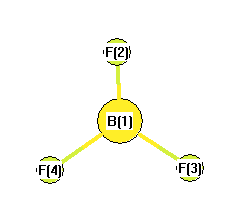Computational Chemistry Comparison and Benchmark DataBase Release 19 (April 2018) Standard Reference Database 101 National Institute of Standards and Technology Home All data for one species Geometry Experimental Calculated Comparisons Bad Calculations Tutorials and Explanations Vibrations Experimental Calculated Scale factors Reactions Entropies Ions List Ions Energy Electron Affinity Proton Affinity Ionization changes point group Experimental One molecule all properties One property a few molecules Geometry Vibrations Energy Electrostatics Reference Data Calculated Energy Optimized Reaction Internal Rotation Orbital Nuclear repulsion energy Correlation Ion Excited State Basis Set Extrapolation Geometry Vibrations Frequencies Zero point energy (ZPE) Scale Factors Bad Calculations Electrostatics Charges Dipole Quadrupole Polarizability Spin Entropy and Heat Capacity Reaction Lookup by property Comparisons Geometry Vibrations Energy Entropy Electrostatics Ion Resources Info on Results Calculations Done Basis functions used I/O files Glossary Conversion Forms Links NIST Links External links Thermochemistry Tutorials Vibrations Entropy Energy Electrostatics Geometry Cost Bad Calculations FAQ Help Units Choose Units Explanations Credits Just show me Summary Using List Recent molecules Molecules Geometry Vibrations Energy Similar molecules Ions, Dipoles, etc. Index of CCCBDB Feedback You are here: Calculated > Energy > Optimized > Energy

# All results from a given calculation for BF3(Borane, trifluoro-)

## using model chemistry: CID/6-31G(2df,p)

### States and conformations

State Conformation minimum conformation conformer description state description
1 1 yes D3H 1A'
Energy calculated at CID/6-31G(2df,p)
hartrees
Energy at 0K-323.898601
Energy at 298.15K
HF Energy-323.215128
Nuclear repulsion energy112.265432
The energy at 298.15K was derived from the energy at 0K and an integrated heat capacity that used the calculated vibrational frequencies.
Vibrational Frequencies calculated at CID/6-31G(2df,p)
Mode Number Symmetry Frequency (cm-1) Scaled Frequency (cm-1) IR Intensities (km mol-1)
1 A1' 938 938 0.00
2 A2" 737 737 113.45
3 E' 1561 1561 409.76
3 E' 1561 1561 409.76
4 E' 512 512 13.29
4 E' 512 512 13.29

Unscaled Zero Point Vibrational Energy (zpe) 2909.9 cm-1
Scaled (by 1) Zero Point Vibrational Energy (zpe) 2909.9 cm-1
See section III.C.1 List or set vibrational scaling factors to change the scale factors used here.
See section III.C.2 Calculate a vibrational scaling factor for a given set of molecules to determine the least squares best scaling factor.
Rotational Constants (cm-1) from geometry optimized at CID/6-31G(2df,p)
ABC
0.35130 0.35130 0.17565

See section I.F.4 to change rotational constant units
Geometric Data calculated at CID/6-31G(2df,p)

Point Group is D3h

Cartesians (Å)
Atom x (Å) y (Å) z (Å)
B1 0.000 0.000 0.000
F2 0.000 1.298 0.000
F3 1.124 -0.649 0.000
F4 -1.124 -0.649 0.000

Atom - Atom Distances (Å)
B1 F2 F3 F4
B11.29761.29761.2976
F21.29762.24762.2476
F31.29762.24762.2476
F41.29762.24762.2476More geometry information
Calculated Bond Angles
atom1 atom2 atom3 angle atom1 atom2 atom3 angle
F2 B1 F3 120.000 F2 B1 F4 120.000
F3 B1 F4 120.000
Electronic energy levels
Charges, Dipole, Quadrupole and Polarizability